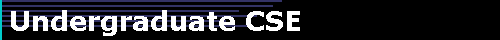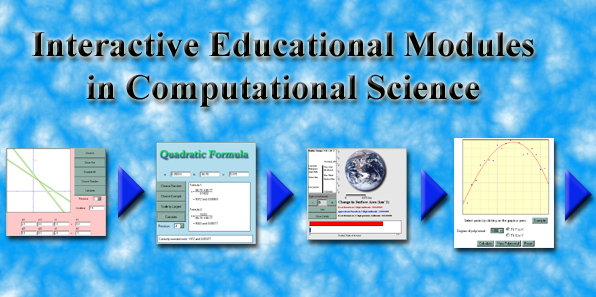"This project is composed of a series of interactive educational modules for learning the basic principles and algorithms of scientific computing."Each module is a Java applet that is accessible through a web browser.  The student selects problem data and algorithm choices interactively and then receives immediate visual feedback on the results, both numerically and graphically.  Topics covered thus far include floating-point arithmetic (precision, rounding error, rounding rules, cancellation, examples of anomalies in summing a series and the quadratic formula), systems of linear equations (graphing solutions, Gaussian elimination, matrix conditioning), least squares data fitting, and polynomial interpolation.  Eventually, we intend to cover all major topics in scientific computing, including eigenvalues, singular values, nonlinear equations, optimization, integration, differential equations, Fourier transforms, and random numbers.  For preliminary versions of modules developed to this date, select "Activities" from the menu above.